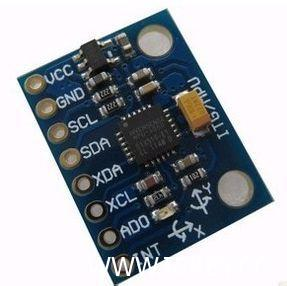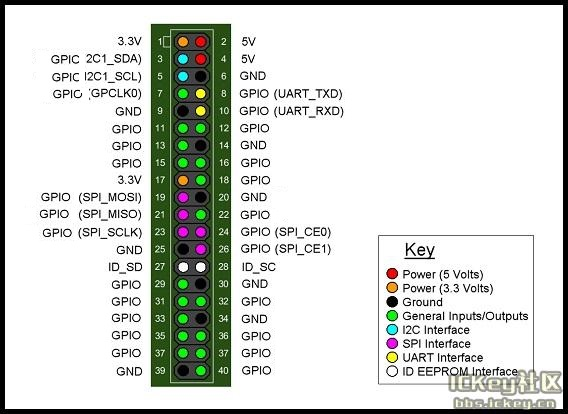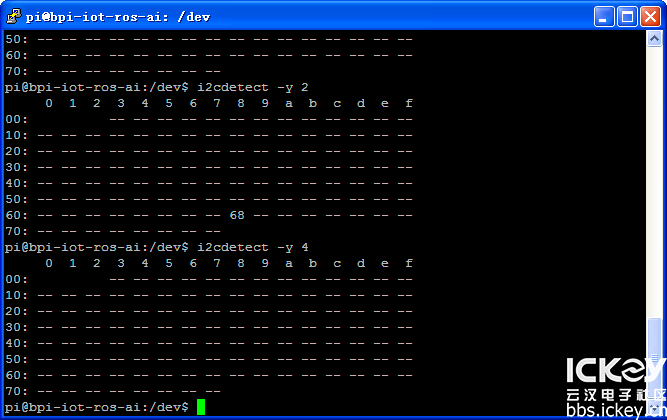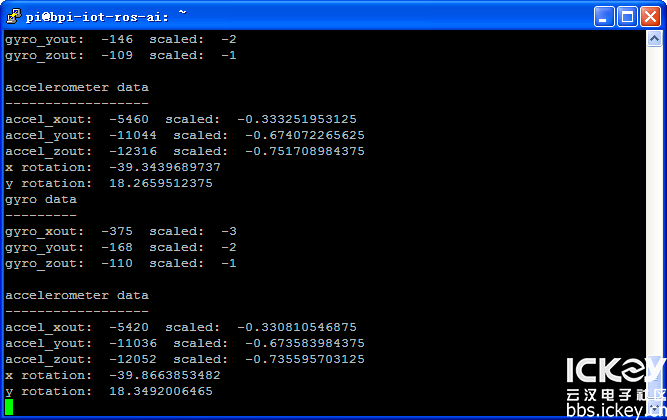# BPI-M2 Berry use GY-521 Six-axis attitude sensor

BPI-M2 Berry use GY-521 Six-axis attitude sensor.

Gy-521 is the 6DOF sensor with the three-axis acceleration and three-axis gyroscope with MPU6050 module. The following author will introduce the method of reading attitude data using I2C.Gy-521 GND VCC SDA SCL was connected to GND VCC SDA SCL of banana pie bpi-m2 Berry development board.Then power the development board and log in to the terminal to enter the following command .i2c-tools and python-smbus

`sudo apt-get install i2c-tools python-smbus`

Then enter the following command to view the I2C interface location.

``````ls /dev
``````then you can see it have i2c-0 i2c-1 i2c-2 i2c-4，

`i2cdetect -y number`From the figure above, you can see gy-521 on i2c-2, and enter the following command to create the python script mp6050.py.

`vi mp6050.py`

Enter the following code

``````import smbus
import time

bus = smbus.SMBus(2)
bus.write_byte_data(0x68, 0x6b, 0)

while True:

gyro_x = ((gyro_data << 8) + gyro_data)
gyro_y = ((gyro_data << 8) + gyro_data)
gyro_z = ((gyro_data << 8) + gyro_data)

accel_x = ((accel_data << 8) + accel_data)
accel_y = ((accel_data << 8) + accel_data)
accel_z = ((accel_data << 8) + accel_data)
print('gyro: x=%05d, y=%05d, z=%05d \t\t accel: x=%05d, y=%05d, z=%05d'%(gyro_x,gyro_y,gyro_z,accel_x,accel_y,accel_z))
time.sleep(1)
``````

After the ESC key is saved, enter the following command to run.

``````python mp6050.py
``````

The following figure shows the printout of the gy-521 raw data.

Press CTRL + C to stop

Next, create a scaled python data script to read mpu-6050.py.

`vi mpu-6050.py`

Enter the following code:

``````import smbus
import math

power_mgmt_1 = 0x6b
power_mgmt_2 = 0x6c

val = (high << 8) + low
return val

if (val >= 0x8000):
return -((65535 - val) + 1)
else:
return val

def dist(a,b):
return math.sqrt((a*a)+(b*b))

def get_y_rotation(x,y,z):

def get_x_rotation(x,y,z):

bus = smbus.SMBus(2)

while True:
print "gyro data"
print "---------"

print "gyro_xout: ", gyro_xout, " scaled: ", (gyro_xout / 131)
print "gyro_yout: ", gyro_yout, " scaled: ", (gyro_yout / 131)
print "gyro_zout: ", gyro_zout, " scaled: ", (gyro_zout / 131)

print
print "accelerometer data"
print "------------------"

accel_xout_scaled = accel_xout / 16384.0
accel_yout_scaled = accel_yout / 16384.0
accel_zout_scaled = accel_zout / 16384.0

print "accel_xout: ", accel_xout, " scaled: ", accel_xout_scaled
print "accel_yout: ", accel_yout, " scaled: ", accel_yout_scaled
print "accel_zout: ", accel_zout, " scaled: ", accel_zout_scaled

print "x rotation: " , get_x_rotation(accel_xout_scaled, accel_yout_scaled, accel_zout_scaled)
print "y rotation: " , get_y_rotation(accel_xout_scaled, accel_yout_scaled, accel_zout_scaled)
time.sleep(1)
``````

Save and enter the following command to run.

`python mpu-6050.py`